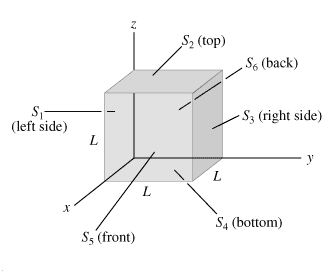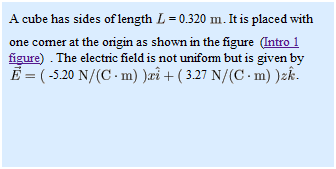# Electric flux through a cube

## Homework StatementE * A

## The Attempt at a Solution

I need to find the electric flux through each face. I am a bit confused. I believe the flux through sides 3 and 1 are zero because those sides are parallel to the electric field. The whole flux through the cube should be 0, I am not sure how to find the electric flux through the other sides because the electric field has 2 components.

Last edited:

Would the angle be Tan(3.27/5.20) for the electric field?

Ok, if I start to write out the area vectors I get.

A1 = -L$$^{2}$$j
A2 = L$$^{2}$$k
A3 = L$$^{2}$$j
A4 = -L$$^{2}$$k
A5 = L$$^{2}$$i
A6 = -L$$^{2}$$i

Now I need to multiple these by the electric field, what is the best way to do it?

anyone?

Use the definition of flux. It is the dot product of the electric field and the outwardly directed area vector. Since the electric field is non-uniform, you will need to do a little calculus. You should notice that the x-coordinate is constant on two faces, and the z-coordinate is constant on two faces.

I am still confused, I haven't really done a problem before with a non-uniform electric field. My textbook doesn't really have any good examples of this.

Ahhh, I think I understand it now.